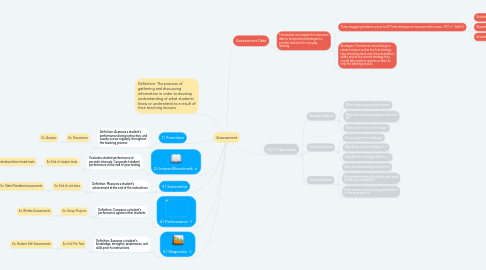# Assessment

Get Started. It's FreeAssessment## 2. 1.) Formative

### 2.1. Definition: Assesses a student's performance during instruction, and usually occurs regularly throughout the teaching process

2.1.1. Ex: Discussions

2.1.1.1. Ex: Quizzes

## 3. 2.) Interim/Benchmark

### 3.1. Evaluates student performance at periodic intervals. Can predict student performance at the end of year testing

3.1.1. Ex: End of chapter tests

3.1.1.1. Ex: Standardized benchmark tests

## 4. 3.) Summative

### 4.1. Definition: Measures a student's achievement at the end of the instructions

4.1.1. Ex: End of unit tests

4.1.1.1. Ex: State Mandated assessments

## 5. 4.) Performance

### 5.1. Definition: Compares a student's performance against other students

5.1.1. Ex: Group Projects

5.1.1.1. Ex: Written Assessments

## 6. 5.) Diagnostic

### 6.1. Definition: Assesses a student's knowledge, strengths, weaknesses, and skills prior to instructions

6.1.1. Ex: Unit Pre-Test

6.1.1.1. Ex: Student Self-Assessments

## 7. Assessment Data

### 7.1. The teacher can analyze the classroom data to find practical strategies to provide solutions for everyday learning.

7.1.1. Three struggling students scores for RTI and strategies to improves their scores. RTI( +/- Std Err)

7.1.1.1. Student 1: 182

7.1.1.2. Student 2: 187

7.1.1.3. Student 3: 193

7.1.2. Strategies: The teachers should begin a reteach session and as the first strategy they should go back over the presentation slides, and as the second strategy they should take practice quizzes or tests to help the learning process

## 8. H.O.T. Questions

### 8.1. Opening Questions

8.1.1. What is the purpose of this lesson?

8.1.2. What does this teaching lesson ask us to do?

8.1.3. What is the theme of the lesson?

### 8.2. Guiding Questions

8.2.1. Do you support this strategy?

8.2.2. Why do we use the strategy of _______?

8.2.3. How does this strategy relate to ________?

### 8.3. Closing Questions

8.3.1. How does this strategy connect to?

8.3.2. If you were planning this lesson plan, what would your strategy be?

8.3.3. How can we use this strategy and relate it to the assessments?